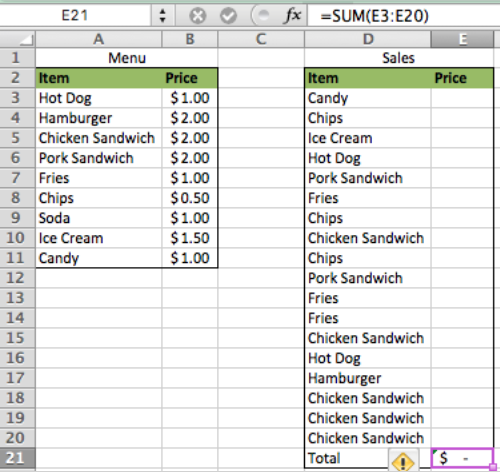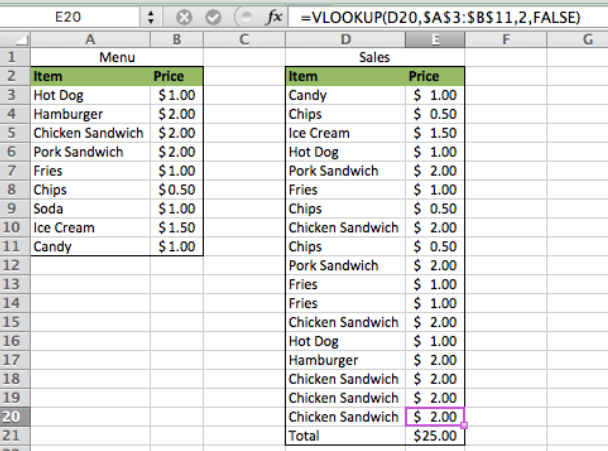Get instant live expert help with Excel or Google Sheets“My Excelchat expert helped me in less than 20 minutes, saving me what would have been 5 hours of work!”

#### Post your problem and you’ll get Expert help in seconds.

Your message must be at least 40 characters
Our professional Expert are available now. Your privacy is guaranteed.

# How to Lock a VLOOKUP from a Table Array

By Locking the VLOOKUP from a table array, we can quickly reference a set of data against multiple lookup values. This tutorial will give you step by step instructions on how to lock the VLOOKUP table

Final formula: `=VLOOKUP(D3,\$A\$3:\$B\$11,2,FALSE)`

## Setting up the Data

In this example the owner of Icy Treats wants to quickly know the total sales for the day. This can be achieved by using a locked VLOOKUP table array.

• First, we set our data up so that the items on the menu are in Column A and the price for each item is in Column B. This will be the table array that we reference in our VLOOKUP function
• Next, create another table by making Column D the items sold for the day and type this formula in cell E21 =sum(E3:E20) This will automatically add the sales prices once we calculate them with the VLOOKUP functionFigure 1 – Setting up the data

Now all we have to do is add our VLOOKUP formula in Column E

## Locking the VLOOKUP

VLOOKUP syntax: `VLOOKUP(Lookup Value, Table Array, Column to Return, Approximate Match [True/False])`

If we were going to be using the table for only one cell, the above syntax would work as planned. However, since we want to test the table on multiple cells, we have to add an extra factor; The Absolute Reference

• An Absolute Reference can be created by typing a “\$” in front of either the row or column of a cell reference. This will allow an Excel user to copy the cell reference to other cells while locking the reference point For example, take \$A\$1. The “\$” in front of the A locks the reference to Column A and the “\$” in front of the 1 locks the reference to row 1. Therefore, the reference is locked to cell A1
• We can take this same approach to a set of data by creating Absolute References to the starting and ending cells of the table array
• In cell E3 type this formula =VLOOKUP(D3,\$A\$3:\$B\$11,2,FALSE)
• Copy the formula in cell E3 and paste it down to cell E20Figure 2 – Locking the VLOOKUP

We can see in this example that the VLOOKUP formula quickly looked at 17 sales and returned the price for each of them by referencing only one table.

Bonus: A keyboard shortcut for creating an Absolute Reference is by pressing the F4 key on your keypad after typing the cell reference

## Instant Connection to an Expert through our Excelchat Service:

Most of the time, the problem you will need to solve will be more complex than a simple application of a formula or function. If you want to save hours of research and frustration, try our live Excelchat service! Our Excel Experts are available 24/7 to answer any Excel question you may have. We guarantee a connection within 30 seconds and a customized solution within 20 minutes.

Are you still looking for help with the VLOOKUP function? View our comprehensive round-up of VLOOKUP function tutorials here.

### Did this post not answer your question? Get a solution from connecting with the expert.Another blog reader asked this question today on Excelchat:
Solution examplesI have a pivot table in which per order (on the rows) stands how much products they ordered per size (on the columns). I want to determine which combinations of quantities of sizes people order. And I want to count these combinations.
Solved by E. W. in 60 minsI have 500 numbers in column A with aproximatly 15digits. In column B I have 5 numbers with 6 digits Can a find a formula wich can transfer all numbers from A column wich start with some 6 digitsa from B column
Solved by V. L. in 32 minsI have a sheet with 3 columns. First column is a code for records in column B (has 563 records). What I need is a formula to show me if what is in column C (has 4400 records) is in column B and if it is to take the code for that record. Example: Column A(code corresponding to column B): 12, 14, 15, 19 Column B(names): asd, adf, ade, aqw Column C(names): akd, adf, ade, anb, wgs I need something that would say, if record in column C (for example adf) is matching record in column B (adf) return the code from column A (the code corresponding to that record in column B),if not return blank.
Solved by C. J. in 8 minsIf the data in A matches the data in C, then I need the data in E to be placed in B
Solved by X. W. in 40 minsI have a workbook with 2 sheets. First sheet has column A which is a device name, then columns B - infinity that have numeric "tags" for that device. Each device can have one or 20 "tags", tags are all numerical. Worksheet 2 has column A which is numeric value and B which is a human readable value of what the tag is. Question is, how do I replace all the tag numbers in worksheet 1 with the actual human readable values is sheet 2?
Solved by T. H. in 16 mins## Subscribe to Excelchat.coAnother blog reader asked this question today on Excelchat: